# Units - math word problems

1. Spherical tank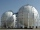The tank of a water tower is a sphere of radius 35ft. If the tank is filled to one quarter of full, what is the height of the water?
2. Tractors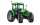Wheel and belt tractors each day plow 8 ha of fields. For plow 76 hectares, the wheel would have to work for 12 days and 8 days with the belt tractor together. How many hectares do each day of each tractor?
3. Two bricks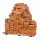One brick weighs 1kg and half a brick, how many weigh two bricks?
4. MorningJana rose in the morning in the quarter to seven. She slept nine and a half hours. When did she go to sleep?
5. Milk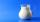At the kindergarten, every child got 1/5 liter of milk in the morning and another 1/8 liter of milk in the afternoon. How many liters were consumed per day for 20 children?
6. HectolitersHow many hectoliters of water is in garden barrel with 90 cm diameter and a height of 1.3 m, if it is filled to 80% of its capacity?
7. Cube cutIn the ABCDA'B'C'D'cube, it is guided by the edge of the CC' a plane witch dividing the cube into two perpendicular four-sided and triangular prisms, whose volumes are 3:2. Determine in which ratio the edge AB is divided by this plane.
8. Collect rain waterThe garden water tank has a cylindrical shape with a diameter of 80 cm and a height of 12 dm. How many liters of water will fit into the tank?
9. Photo frameA square shape photo with a side length of 20 cm is framed by a 4 cm wide bar. Find the external frame size of this photo (the frame is from all sides)
10. Perimeter of baseThe circumference of the base of the rotating cylinder is same as its height. What is the diameter and height of this cylinder with volume 1 liter?
11. PexesoDrawing paper has dimensions of 60cm and 840mm. Pupils have to divide it into squares so that they can make a pexeso. What dimension must squares have if their side is to be larger than 3cm and less than 10cm?
12. If theIf the tangent of an angle of a right angled triangle is 0.8. Then its longest side is. .. .
13. Two airportsTwo airports are 2690 km away. From the first airport, the airplane flies at a speed of 600 km/h, from a second airplane at a speed of 780 km/h. When will they meet if they flew at 10:00? How far from the first airport?What overload in g (g-force) has passed the pilot if he accelerated from 0 to 600 km/h in 3 seconds?
15. Diameter of a cylinderThe diameter of the cylinder is 42 cm. How many times does the cylinder turn on a 66m long track?
16. Mixing waterThe 30-liter container should we fill with water at 60 degrees Celsius. How many liters of water 80 degrees C hot and how many liters of water 20 degrees Celsius warm we have to mix?
17. The sidesThe sides of a rectangle are in a ratio of 2:3, and its perimeter is 1 1/4 inches. What are the lengths of its side? Draw it.
18. Volume of cone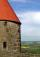Find the volume of a right circular cone-shaped building with a height of 9 cm and a radius base of 7 cm.
19. GrandmotherMom walked out to visit her grandmother in a neighboring village 5km away and moved at a speed of 4km/h. An hour later, father drove down the same road at an average speed of 64km/h. 1) How long will take to catch mom die? 2) What is the approximate dis
20. Clock's gears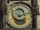In the clock machine, three gears fit together. The largest has 168 teeth, the middle 90 teeth, and the smallest 48 teeth. The middle wheel turns around its axis in 90 seconds. How many times during the day do all the gears meet in the starting position?

Do you have an interesting mathematical word problem that you can't solve it? Enter it, and we can try to solve it.

To this e-mail address, we will reply solution; solved examples are also published here. Please enter the e-mail correctly and check whether you don't have a full mailbox.

Please do not submit problems from current active competitions such as Mathematical Olympiad, correspondence seminars etc...